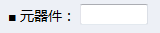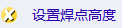2015-05-07 01:33

# JavaScript点击按钮弹出一个可操作窗口或表格• 点赞
• 写回答
• 关注问题
• 收藏
• 邀请回答

#### 1条回答默认 最新

•已采纳
`````` 元器件：<input type="text" id="t" value="a;b;c;d;e" /><br />
<a href="javascript:setHeight()">设置焊点高度</a>
<div id="dvHeight"></div>
<script>
function setHeight() {
var t = document.getElementById('t');
if (t.value == '') { alert('请输入元器件！'); t.focus(); return }
var arr = t.value.split(';'), s = '<table>';
for (var i = 0; i < arr.length; i++) {
s += '<tr><td>' + arr[i] + ':</td><td><input type="text"/></td></tr>';
}
s += '<tr><td colspan="2"><input type="button" value="获取高度" onclick="getHeight()"></td></tr></table>';
document.getElementById('dvHeight').innerHTML = s;
}
function getHeight() {
var ipt = document.getElementById('dvHeight').getElementsByTagName('input'), s = '';
for (var i = 0; i < ipt.length; i++) {
if (ipt[i].type == 'text') {
if (ipt[i].value == '') { alert('请输入高度！'); ipt[i].focus(); return }
s += ';' + ipt[i].value;
}
}
s = s.substring(1);